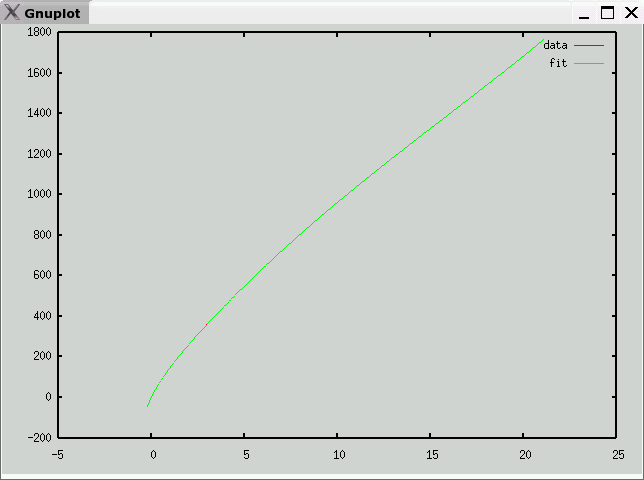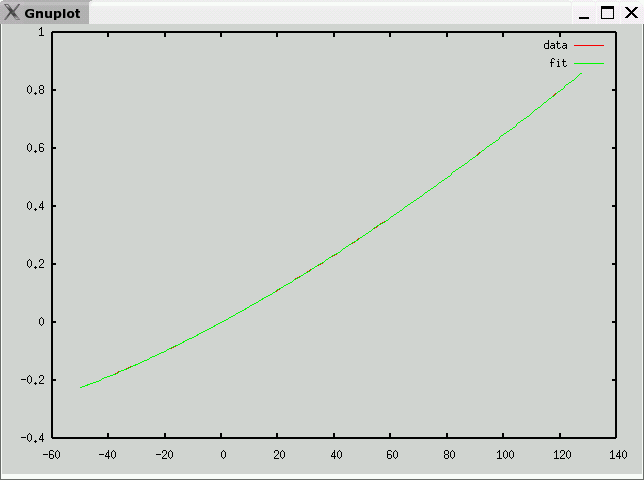# Type R Thermocouple Data and Polynomial

• Description
Pt(13%)-Rh / Pt
• Data source
National Institute of Science and Technology (NIST)
• Raw Data
• Octave format
• Curve fit
• mV -> temperature (C)
e is maximum error (in degrees C)
v1 andf v2 partition the domain into three segments, each with a polynomial fit
c is vector of coefficients: a10, a9, ... a0
Note: Each column of c corresponds to one range (<v1,v1 to v2, >v2)

`e = 0.12959v1 = 1.9420v2 = 12.687c =   -2.9783e+00   -1.1534e-08   -1.6007e-06    2.8649e+01   1.0740e-06    2.7281e-04   -1.1740e+02   -4.2822e-05   -2.0812e-02    2.6821e+02   9.8167e-04    9.3604e-01   -3.7755e+02   -1.4596e-02   -2.7491e+01    3.4710e+02   1.4984e-01    5.5096e+02   -2.2683e+02   -1.0929e+00   -7.6321e+03    1.3035e+02   5.6847e+00    7.2164e+04   -9.3397e+01   -2.2016e+01   -4.4578e+05    1.8887e+02   1.5152e+02    1.6248e+06   -1.6514e-02    1.1243e+01  -2.6531e+06 `• temperature -> mV (range -127C-127C)
e is maximum error (in mV)
c is vector of coefficients: a10, a9, ... a0

`e = 0.00057212c =   -2.6125e-22    5.7164e-20    4.5413e-18   -1.9955e-15    1.3374e-13    3.0986e-12   -4.6068e-10   -1.9974e-08    1.4302e-05    5.2867e-03    3.1372e-05 `Previous page: Type N
Next page: Type S